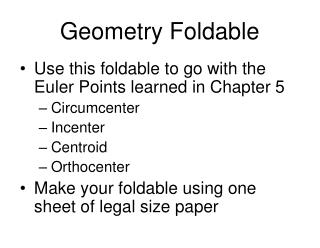DownloadDownload PresentationGeometry Foldable

# Geometry Foldable

Download Presentation## Geometry Foldable

- - - - - - - - - - - - - - - - - - - - - - - - - - - E N D - - - - - - - - - - - - - - - - - - - - - - - - - - -
##### Presentation Transcript

1. Geometry Foldable • Use this foldable to go with the Euler Points learned in Chapter 5 • Circumcenter • Incenter • Centroid • Orthocenter • Make your foldable using one sheet of legal size paper

2. Circumcenter – where the 3 perpendicular bisectors cross Is the center of a circle that goes around the outside of a triangle and touches only the vertices

3. Incenter – where the 3 angle bisectors cross Is the center of a circle that goes inside a triangle and touches the sides of the triangle at only one point

4. Centroid – the balance point where the 3 medians of the triangle cross A median goes from the midpoint of one side to the vertex opposite

5. Orthocenter – where the 3 altitudes of the triangle cross An altitude is measured perpendicular to a side and going to the vertex across

6. For a right triangle the circumcenter is always on the hypotenuse and the orthocenter is always at the 90º angle. For all triangles the incenter and centroid are always inside the triangle